This example shows how to use Simulink® blocks and helper functions provided by Robust Control Toolbox™ to specify and analyze uncertain systems in Simulink and how to use these tools to perform Monte Carlo simulations of uncertain systems.

### Introduction

The Simulink model `usim_model` consists of an uncertain plant in feedback with a sensor:

```open_system('usim_model') ```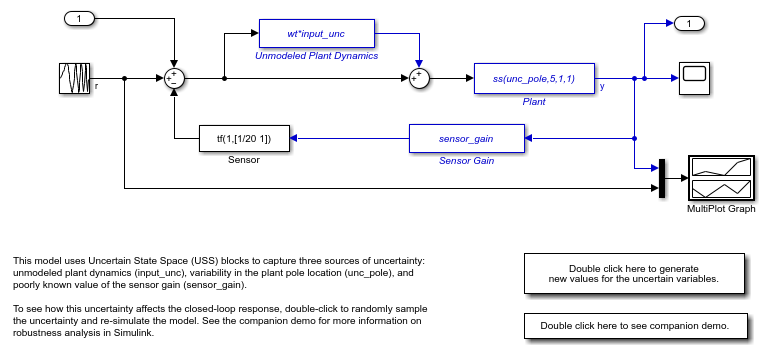The plant is a first-order model with two sources of uncertainty:

• Real pole whose location varies between -10 and -4

• Unmodeled dynamics which amount to 25% relative uncertainty at low frequency rising to 100% uncertainty at 130 rad/s.

The feedback path has a cheap sensor which is modeled by a first-order filter at 20 rad/s and an uncertain gain ranging between 0.1 and 2. To specify these uncertain variables, type

```% First-order plant model unc_pole = ureal('unc_pole',-5,'Range',[-10 -4]); plant = ss(unc_pole,5,1,1); % Unmodeled plant dynamics input_unc = ultidyn('input_unc',[1 1]); wt = makeweight(0.25,130,2.5); % Sensor gain sensor_gain = ureal('sensor_gain',1,'Range',[0.1 2]); ```

### Simulink Blocks for Uncertainty Modeling and Analysis

The `RCTblocks` library contains blocks to model and analyze uncertainty effects in Simulink. To open the library, type

```open('RCTblocks') ```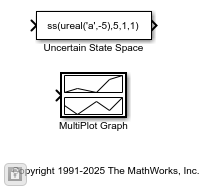The `Uncertain State Space` block lets you specify uncertain linear systems (USS objects). `usim_model` contains three such blocks which are highlighted in blue. The dialog for the "Plant" block appears below.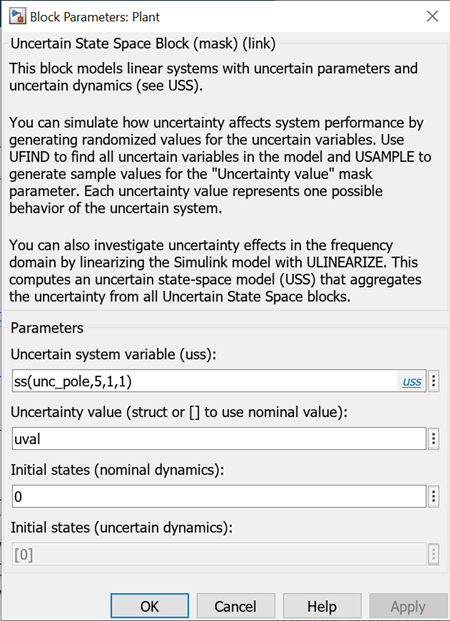In this dialog box,

• The "Uncertain system variable" parameter specifies the uncertain plant model (first-order model with uncertain pole `unc_pole`).

• The "Uncertainty value" parameter specifies values for the block's uncertain variables (`unc_pole` in this case).

`uval` is a structure whose field names and values are the uncertain variable names and values to use for simulation. You can set `uval` to `[]` to use nominal values for the uncertain variables or vary `uval` to analyze how uncertainty affects the model responses.

The `MultiPlot Graph` block is a convenient way to visualize the response spread as you vary the uncertainty. This block superposes the simulation results obtained for each uncertainty value.

### Monte Carlo Simulation of Uncertain Systems

To easily control the uncertainty value used for simulation, `usim_model` uses the same "Uncertainty value" `uval` in all three `Uncertain State Space` blocks. Setting `uval` to `[]` simulates the closed-loop response for the nominal values of `unc_pole`, `input_unc`, and `sensor_gain`:

```uval = []; % use nominal value of uncertain variables sim('usim_model',10); % simulate response ```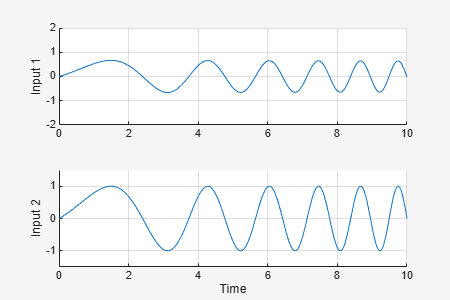To analyze how uncertainty affects the model responses, you can use the `ufind` and `usample` commands to generate random values of `unc_pole`, `input_unc`, and `sensor_gain`. First use `ufind` to find the `Uncertain State Space` blocks in `usim_model` and compile a list of all uncertain variables in these blocks:

```[uvars,pathinfo] = ufind('usim_model'); uvars % uncertain variables ```
```uvars = struct with fields: input_unc: [1x1 ultidyn] sensor_gain: [1x1 ureal] unc_pole: [1x1 ureal] ```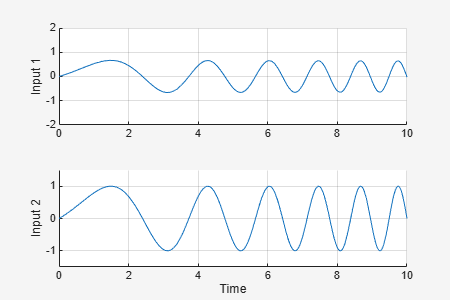```pathinfo(:,1) % paths to USS blocks ```
```ans = 3x1 cell array {'usim_model/Plant' } {'usim_model/Sensor Gain' } {'usim_model/Unmodeled Plant Dynamics'} ```

Then use `usample` to generate uncertainty values `uval` consistent with the specified uncertainty ranges. For example, you can simulate the closed-loop response for 10 random values of `unc_pole`, `input_unc`, and `sensor_gain` as follows:

```for i=1:10; uval = usample(uvars); % generate random instance of uncertain variables sim('usim_model',10); % simulate response end ```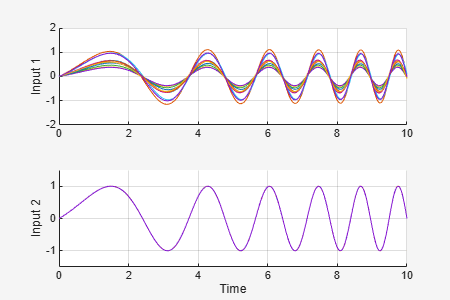The `MultiPlot Graph` window now shows 10 possible responses of the uncertain feedback loop. Note that each `uval` instance is a structure containing values for the uncertain variables `input_unc`, `sensor_gain`, and `unc_pole`:

```uval % sample value of uncertain variables ```
```uval = struct with fields: input_unc: [1x1 ss] sensor_gain: 0.5893 unc_pole: -4.9557 ```

### Randomized Simulations

If needed, you can configure the model to use a different uncertainty value `uval` for each new simulation. To do this, add `uvars` to the Base or Model workspace and attach the `usample` call to the model InitFcn:

```bdclose('usim_model'), open_system('usim_model') % Write the uncertain variable list in the Base Workspace evalin('base','uvars=ufind(''usim_model'');') % Modify the model InitFcn set_param('usim_model','InitFcn','uval = usample(uvars);'); % Simulate ten times (same as pressing "Start simulation" ten times) for i=1:10; sim('usim_model',10); end % Clean up set_param('usim_model','InitFcn',''); ```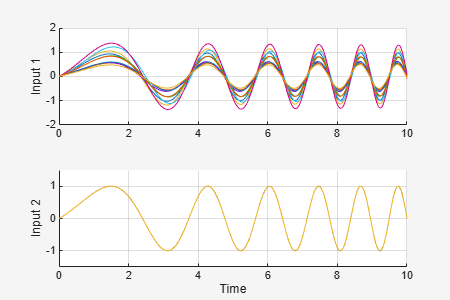Again the `MultiPlot Graph` window shows 10 possible responses of the uncertain feedback loop.

### Linearization of Uncertain Simulink Models

If you have Simulink Control Design™, you can use the same workflow to linearize and analyze uncertain systems in the frequency domain. For example, you can plot the closed-loop Bode response for 10 random samples of the model uncertainty:

```clear sys wmax = 50; % max natural frequency for unmodeled dynamics (input_unc) for i=1:10; uval = usample(uvars,1,wmax); sys(:,:,i) = linearize('usim_model'); end bode(sys) title('Ten linearizations of usim\_model'); ```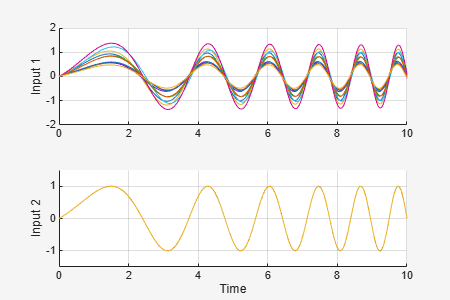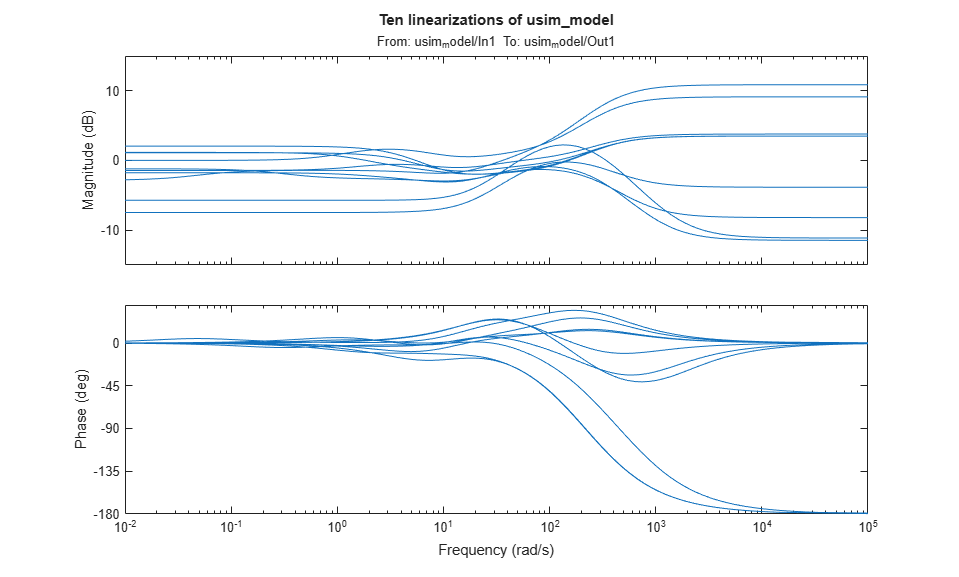If the operating point is independent of the uncertain variables, a faster approach is to compute an uncertain linearization (USS object) in one shot using the `ulinearize` command:

```usys = ulinearize('usim_model') ```
```usys = Uncertain continuous-time state-space model with 1 outputs, 1 inputs, 3 states. The model uncertainty consists of the following blocks: input_unc: Uncertain 1x1 LTI, peak gain = 1, 1 occurrences sensor_gain: Uncertain real, nominal = 1, range = [0.1,2], 1 occurrences unc_pole: Uncertain real, nominal = -5, range = [-10,-4], 1 occurrences Type "usys.NominalValue" to see the nominal value, "get(usys)" to see all properties, and "usys.Uncertainty" to interact with the uncertain elements. ```You can then sample the uncertain state-space model `usys` to generate a similar Bode plot:

```bode(usample(usys,10,wmax)) title('Ten linearizations of usim\_model'); ```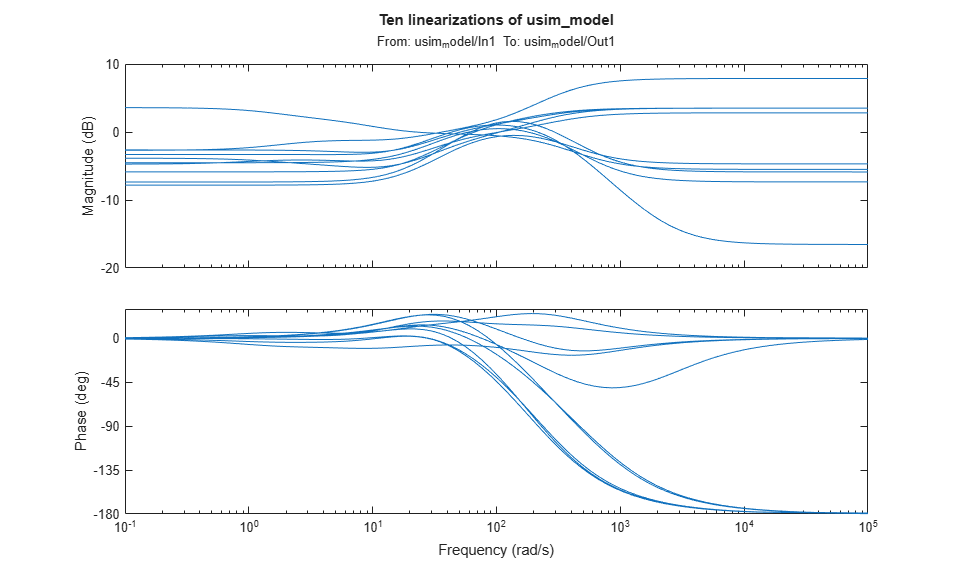## SupportGet trial now